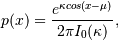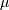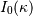# numpy.random.vonmises¶

numpy.random.vonmises(mu, kappa, size=None)

Draw samples from a von Mises distribution.

Samples are drawn from a von Mises distribution with specified mode (mu) and dispersion (kappa), on the interval [-pi, pi].

The von Mises distribution (also known as the circular normal distribution) is a continuous probability distribution on the unit circle. It may be thought of as the circular analogue of the normal distribution.

Parameters: mu : float Mode (“center”) of the distribution. kappa : float Dispersion of the distribution, has to be >=0. size : int or tuple of ints, optional Output shape. If the given shape is, e.g., (m, n, k), then m * n * k samples are drawn. Default is None, in which case a single value is returned. samples : scalar or ndarray The returned samples, which are in the interval [-pi, pi].

scipy.stats.distributions.vonmises
probability density function, distribution, or cumulative density function, etc.

Notes

The probability density for the von Mises distribution iswhereis the mode andthe dispersion, andis the modified Bessel function of order 0.

The von Mises is named for Richard Edler von Mises, who was born in Austria-Hungary, in what is now the Ukraine. He fled to the United States in 1939 and became a professor at Harvard. He worked in probability theory, aerodynamics, fluid mechanics, and philosophy of science.

References

 [R270] Abramowitz, M. and Stegun, I. A. (Eds.). “Handbook of Mathematical Functions with Formulas, Graphs, and Mathematical Tables, 9th printing,” New York: Dover, 1972.
 [R271] von Mises, R., “Mathematical Theory of Probability and Statistics”, New York: Academic Press, 1964.

Examples

Draw samples from the distribution:

>>> mu, kappa = 0.0, 4.0 # mean and dispersion
>>> s = np.random.vonmises(mu, kappa, 1000)


Display the histogram of the samples, along with the probability density function:

>>> import matplotlib.pyplot as plt
>>> from scipy.special import i0
>>> plt.hist(s, 50, normed=True)
>>> x = np.linspace(-np.pi, np.pi, num=51)
>>> y = np.exp(kappa*np.cos(x-mu))/(2*np.pi*i0(kappa))
>>> plt.plot(x, y, linewidth=2, color='r')
>>> plt.show()


#### Previous topic

numpy.random.uniform

#### Next topic

numpy.random.wald